# CBSE Class 10 Maths Chapter 12 Areas Related to Circles Objective Questions

CBSE Class 10 Maths Chapter 12 Areas Related to Circles Objective Questions covers the concepts of the perimeter (circumference) and area of a circle. The chapter also introduces  CBSE Class 10 students to parts of the circle, their measurements, and areas of plane figures. We have formulated these CBSE Class 10 Maths Chapter 12 – Areas Related to Circles Objective Questions based on the new exam pattern and have categorised them topic-wise for the students to prepare well for the board exams.

Students can now prepare ahead for the exams with the help of these chapter-wise CBSE Class 10 Maths Objective Questions that we have compiled.

### Sub-Topics Covered in Chapter 12

Find below the sub-topics that are covered in this chapter.

12. 1 Introduction (5 MCQs)

12.2 Visualisations (5 MCQs)

12.3 Areas of Sector and Segments Related to Circles (3 MCQs)

12.4 Areas of a Combination of Plane Figures (3 MCQs)

12.5 Arc Length (1 MCQ)

12.6 Perimeter and Areas Related to Circles (3 MCQs)

Students can download the PDF given below to access the topic-wise objective questions to prepare well for the board exam.

## Download CBSE Class 10 Maths Chapter 12 – Areas Related to Circles Objective Questions

### Introduction

1. Find the area of the shaded region where ABC is a quadrant of radius 5 cm and a semicircle is drawn with BC as diameter.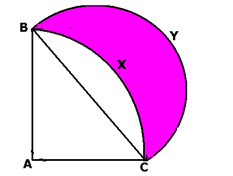1. 8.8 cm2
2. 7.14 cm2
3. 12.5 cm2
4. 19.64 cm2

Solution: Area of the shaded region = Area of the semicircle – Area of the segment of the sector BAC

Area of the semicircle with BC as diameter =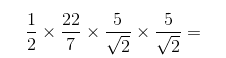19.64cm2

Area of segment = Area of the quadrant – Area of ΔABC

= 90/360 × 22/7 × 52– ½ × 5 × 5=19.64-12.5=7.14 cm2….. (ii)

Area of the shaded region = (i) – (ii) = 19.64 – 7.14 = 12.5 cm2

1. The area of the shaded portion in the following figure is equal to the area of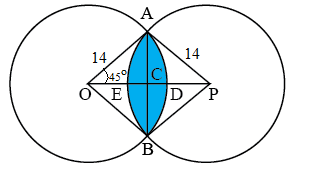2. segment AEBA

Solution: The area of the shaded region will be the sum of the areas of the segments of the circles that form the region. In this case, it is segments ADBA and AEBA.

1. Consider point A on the circle of radius 7/π cm, as shown in the figure. A ball on point A moves along the circumference until it reaches point B. The tangent at B is parallel to the tangent at A. What is the distance travelled by the ball? (Consider the ball to be a point object)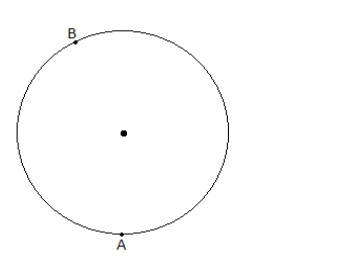Note: Point B in the diagram may not represent its actual position.

1. 3.5 cm
2. 7 cm
3. 14 cm
4. 28 cm

Solution: Given that the tangent at point B is parallel to the tangent at point A. Points A and B would be as follows: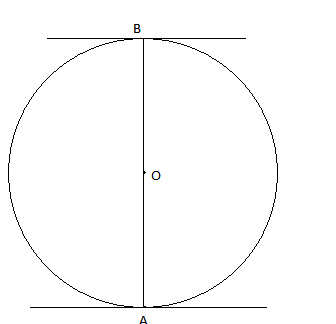The tangents at A and B are parallel, so the radii through A and B should be parallel to each other since the radius is perpendicular to the tangent at the point of contact. Since the radius passes through the centre of the circle, the radii through A and B must lie on the same straight line. Line AB will be the diameter of the circle.

Now, A and B are on two ends of the diameter of the circle. The distance travelled along the circle from A to B will be half the circumference of the circle.

Now, circumference

= 2πr=2×π×7/π=14cm

Distance = 1/2×circumference = 1/2×14cm = 7 cm.

1. A pendulum swings through an angle of 30∘ and describes an arc 8.8 cm in length. Find the length of the pendulum in cm.
1. 16.8 cm
2. 17.3 cm
3. 15.1 cm
4. 14.5 cm

Solution: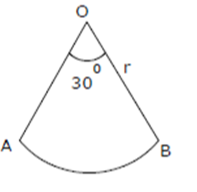Let r be the length of the pendulum

Length of arc = 8.8 cm

∠AOB = 30

Length of an arc of a sector of an angle θ = (θ/360) × 2πr

Length of arc = (θ/360) ×2πr

8.8= (30/360) × 2 × (22/7) × r

r= (8.8×21)/11 = 16.8 cm

1. There is a circular swimming pool with a centre O. The radius of the pool is 5 m. There are 2 points on the wall of the pool separated by a distance of 7 m. These 2 points are named A and B. A rope is attached between A and B. This rope separates the shallow section of the pool from the deep section of the pool. The shallow section is the smaller section. Which of the following statements is true?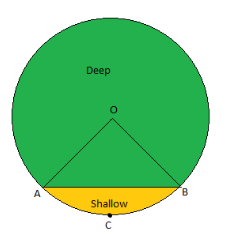1. The shallow section is an arc.
2. The area of the circle between OA and OB is an arc.
3. The shallow section is a segment
4. The shallow section is a sector

Answer: (C) The shallow section is a segment

Solution: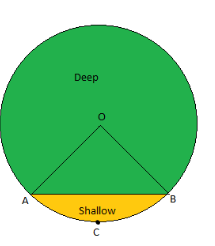The segment is that part of a circle that is made by a line and a connecting arc. Segments touch any two points in a circle. So here, the shallow section is a segment.

### Visualisations

1. In the figure, the area of the portion in orange colour is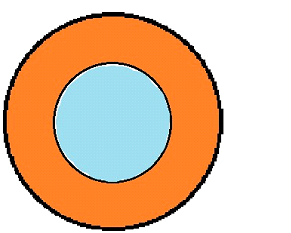1. Area of the outer circle + Area of the inner circle
2. Area of the outer circle – Area of the inner circle
3. Area of the inner circle – Area of the outer circle
4. Area of the outer circle

Answer: (B) Area of the outer circle – Area of the inner circle

Solution: (Area of the outer circle – Area of the inner circle) gives the area of the orange-coloured region in the above figure.

1. Given below is a combination figure of square ABCD of side 26 cm and four circles. Find the area of the shaded region.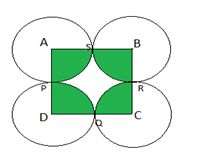1. 530.64 cm2
2. 402.83 cm2
3. 360 cm2
4. 480.53 cm2

Solution: The given figure forms four sectors:

Area of a sector of angle Θ= Θ/360 × πr2

Area of one sector APS = (90/360) × π ×132= 132.66 cm2

Total area of shaded region = Area of four sectors=4 × 132.66 cm= 530.64 cm2

1. In the given figure, a circle is inscribed in a trapezium of height 14 cm, and lengths of parallel sides are 25 cm and 40 cm. What is the area of the shaded region?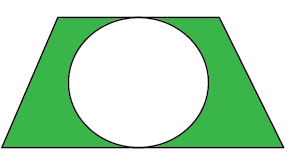1. 455 sq cm
2. 154 sq cm
3. 509 sq cm
4. 301 sq cm

Solution: Area of the shaded region = Area of the trapezium – Area of the circle

Area of trapezium =1/2 × height × sum of parallel sides

=1/2×14× (25+40) = 455 sq cm

Area of the circle = π×r2= (22/7) ×7×7 = 154 sq cm

Hence, area of the shaded region = 455 – 154 = 301 sq cm

1. In the figure below, the radius of the outer circle is 18 cm, and the radius of the inner circle is 7 cm. Then, what is the area of the region between the outer and the inner circles?
1. 361 π cm2
2. 133 cm2
3. 192.5 cm2
4. 275 π cm2

Solution: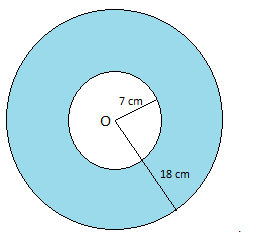Area of the region in between outer and inner circle = Area of the outer circle – Area of the inner circle

Area of the outer circle = π (18)2 = 324 πcm2

Area of the inner circle = π (7)= 49 πcm2

So, the area of the required region = 324 π – 49π = 275 πcm2.

1. A stadium is circular in shape. Within the stadium, some areas have been allotted for a hockey court and a javelin range, as given in the figure. Assume the shape of the hockey court and the javelin range to be square and triangle, respectively. The curators would like to accommodate a few more sports in the stadium. Help them by measuring the unallocated region within the stadium (the radius of the stadium is 200 mts.).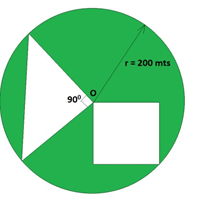1. 40000π m2
2. 40000(π−1) m2
3. 20000(π−1) m2
4. 20000π m2

Solution: We need to find the unallocated area within the stadium.

The unallocated area should be = The total circular area of the stadium – The area of the hockey court – Area of the javelin range.

The area of the circular stadium = π×r2

= π×2002

= 40000π

Regarding the area of the hockey court (square), we know that the radius of the stadium forms the diagonal of the hockey court.

Therefore, the sides of the hockey court will be

$$\begin{array}{l}a=\frac{r}{\sqrt{2}}\end{array}$$
(applying Pythagoras theorem in the square).

Then, the area of the square = a2

$$\begin{array}{l}=(\frac{r}{\sqrt{2}})^{2}\end{array}$$

= r2/ 2

= 2002/2

20000 m2

The javelin range is a right-angled triangle

Area of the right triangle = ½ × r × r

= ½ × 200 × 200

= 20000 m2

Therefore, the unallocated area in the stadium = Total area of the stadium – Area of the hockey court – Area of the javelin range

= 40000π−20000−20000

= 40000(π−1) m2

Hence, the unallocated area within the stadium is 40000(π−1) m2.

### Areas of Sector and Segments Related to Circles

1. There is a circle of diameter 10 cm. A chord of length 6 cm is drawn inside the circle. What is the distance between the centre and this chord in cm?
1. 1.5
2. 2
3. 4
4. 3

Solution: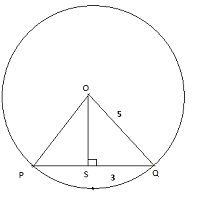Radius = 1/2 of diameter = 5 cm

OS is drawn from O and is perpendicular to chord PQ and bisects it.

So, SQ = 3 cm

Hence, the length of OS is the distance between O and PQ.

OQ2= OS2 + SQ2

52 = OS2+ 32

⇒ OS = 4 cm.

Hence, the distance between the centre and the chord is 4 cm.

1. Find the area of the shaded region.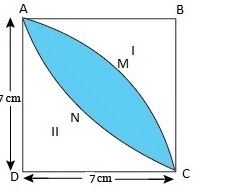1. 24 cm2
2. 25 cm2
3. 28 cm2
4. 21 cm2

Solution: Area of square ABCD = 7×7 =49 cm2

Area of AMCDA = ¼ × π× 72 = 1/4×22/7×72 = 38.5 cm2

Area of ADCNA = Area of AMCDA = 38.5 cm2

Area of shaded region = Area of ADCNA + Area of AMCDA – Area of square ABCD

→2× Area of ADCNA – Area of square ABCD

= 2×38.5 – 49 = 28 cm2

1. Find the area of the shaded region.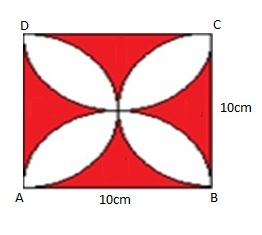1. 38 cm2
2. 57 cm2
3. 43 cm2
4. 62 cm2

Solution: Divide the square ABCD into four equal parts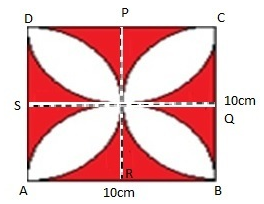And take one part which is given by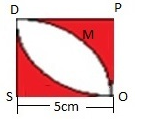Area of the shaded region in this part = Area of square DPOS – Area of the unshaded region

And area of PDMO = 52 – ¼ × π×r2 = 25− ¼ ×3.14×25 = 5.375 cm2

Therefore, the area of the shaded region in square DPOS = 5.375×2 = 10.75 cm2

As DPOS is a quarter of square ABCD,

The total area of the shaded region = 4×10.75=  43 cm2

### Areas of Combination of Plane Figures

1. If a square with side ‘a’ is inserted within a circle such that the corners coincide with the circumference of the circle with diameter ‘d’, find the relation between ‘a’ and ‘d’.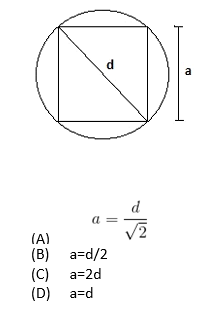Solution: We are asked to find the relation between the diameter of the circle and the side of the square.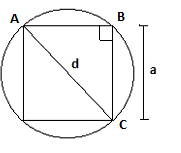Let us consider the triangle ABC,

$$\begin{array}{l}(\angle ABC = 90^\circ)\end{array}$$

Therefore, we can consider triangle ABC as a right-angled triangle with sides = ‘a’ and hypotenuse =‘d’.

Applying the Pythagoras equation, we get d2=a2+a2.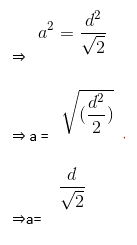Therefore, the relation between the sides of the square and the diameter of the circle is, a = d/√2.

1. If an equilateral triangle is drawn inside a circle such that the circle is the circum-circle of the triangle, find the relation between the length of the triangle and the radius of the circle.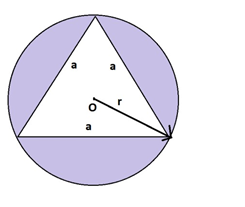1. √3 × r
2. $$\begin{array}{l}\frac{r}{\sqrt{3}}\end{array}$$
3. 3 r
4. r/3

Solution: Here, we need to find the relation between the circle radius and the side length of the equilateral triangle.

Let us consider the radius of the circle is ‘r’ and the side length of the triangle is ‘a’.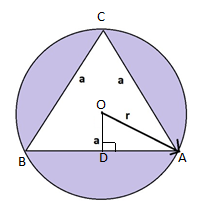Construction: Join OA. Draw OD perpendicular to AB. Since OD is perpendicular to AB, it bisects the chord. Therefore, AD = 1/2×AB

In the triangle AOD,

∠AOD=1/2∠AOB      (triangle AOB is an isosceles triangle)

Because the angle is subtended by a chord A, the centre O is double the angle subtended by the chord at any point on the circumference.

∠AOB=2×∠ACB       ∠ACB is the internal angle of the equilateral triangle)

= 2×60

=120   ​

Therefore, ∠AOD=12×120   ​

∠AOD=60

Applying trigonometric properties in rt triangle AOD,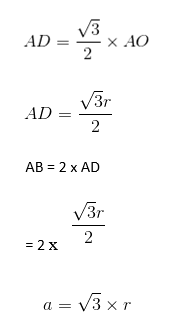Thus, the relation between the side of the equilateral triangle and the radius of the circumcircle is, a = √3 × r.

1. In the figure below, AB and CD are two diameters of a circle (with centre O) perpendicular to each other, and OD is the diameter of the smaller circle. If OA = 7 cm, find the area of the shaded region.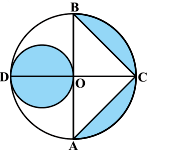1. 65.5 cm2
2. 66.5 cm2
3. 67.5 cm2
4. 68.5 cm2

Solution: Radius of the larger circle, R = 7 cm

The radius of the smaller circle, r=7/2cm

Height of ΔBCA=OC=7 cm

Base of ΔBCA=AB=14 cm

Area of ΔBCA=1/2×AB×OC=1/2×7×14=49 cm2

Area of larger circle =π×R2=22/7×72=154cm2

Area of larger semicircle =154/2cm2=77 cm2

Area of smaller circle =π × R2=22/7×7/2×7/2=77/2cm2

Area of the shaded region = Area of the larger circle – Area of the triangle – Area of larger semicircle + Area of the smaller circle

Area of the shaded region =(154−49−77+77/2)cm2

= 133/2cm2 = 66.5 cm2

Thus, the area of the shaded region is 66.5 cm2.

### Arc Length

1. A wire is bent to form a circle of radius 7 cm. From the resulting shape, a chunk of the wire is cut off, and the wire cut off measures 4 cm in length. The length of the remaining wire is
1. 45 cm
2. 50 cm
3. 40 cm
4. 42 cm

Solution: The length of the wire = circumference of the circle with a radius of 7 cm

=2×π×r=2×22/7×7 = 44 cm.

When a wire of length 4 cm is cut off, the length of the wire remaining = Circumference – length of arc cut off = 44 – 4 = 40 cm.

### Perimeter and Areas Related to Circles

1. If the perimeter and the area of a circle are numerically equal, then the radius of the circle is
1. 3 units
2. 4 units
3. π units
4. 2 units

Solution: Let the radius of the circle be r.

∴ The perimeter of the circle = Circumference of the circle = 2πr

∴ Area of the circle = πr2

Given: Area = Perimeter

⇒2πr = πr2

⇒2 = r

Thus, the radius of the circle is 2 units.

1. In the given figure, OACB is a quadrant of a circle. The radius OA = 3.5 cm, OD = 2 cm. Calculate the area of the shaded region.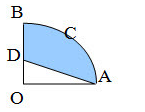1. 5.125 cm2
2. 6.5 cm2
3. 7 cm2
4. 6.125 cm2

Solution: Area of a quadrant of a circle = 1/4 × Area of the circle.

∴ Area of the shaded region = 1/4× Area of the circle – Area of the triangle.

⟹ Area of the shaded region = 1/4×πr2   −1/2base×height

⟹ Area of the shaded region = 1/4×22/7×3.5×3.5 −1/2×3.5×2

⟹ Area of the shaded region = 9.625 – 3.5 = 6.125 cm2

1. The shaded area in the adjoining figure, between the circumferences of two concentric circles, is 346.5 cm2. The circumference of the inner circle is 88 cm. Calculate the radius of the outer circle. [Take π=22/7]
1. 35 cm
2. 32 cm
3. 17.5 cm
4. 16.5 cm

Solution: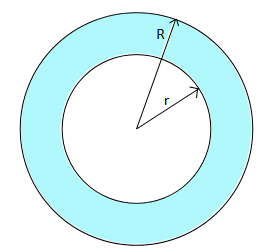Let the radii of the inner circle and outer circle be r and R, respectively.

Then, 2 × 22/7 × r=88

∴ r = 14 cm.

Area of the inner circle = 22/7 × 14 × 14cm2 = 616cm2

∴ Area of the outer circle = (616 + 346) cm2 = 962.5 cm2

Then, 22/7×R2=962.5

⟹R2=962.5×7/22=6.25×7×7

⟹R=2.5×7 = 17.5 cm

Thus, the radius of the outer circle is 7.5 cm.

Class 10 Objective Questions from Chapter 12 Areas Related to Circles, provided here, are extremely useful for the CBSE  Class 10 students to prepare for the board exams and also to revise the important MCQs from this chapter in the most efficient way.

For extra practice, students can refer to the extra questions provided below.

### CBSE Class 10 Maths Chapter 12 Extra Questions

1. Given that the angle of the sector is 60° and the radius is 3.5 cm, calculate the length of the arc.
(a) 3.5 cm
(b) 3.66 cm
(c) 3 cm
(d) 3.8 cm

2. If the diameter of a circle is 21 cm, then calculate its area.
(a) 346.5 cm²
(b) 37.68 cm²
(c) 18.84 cm²
(d) 19.84 cm²

Be sure to visit BYJU’S for more study materials for CBSE Class 10 students in order to practise rigorously for the board exam.